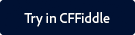Note:

You can find the CFFiddle demo of this function and other spreadsheet functions as part of a project that is shared with you.

Click the button below to launch CFFiddle.To copy the project in your workspace in CFFiddle, follow the steps below:

1. Log in with your Gmail or Facebook credentials.
2. Navigate to the project in the left pane.
3. Once you make some changes in any cfm in the project, a pop up displays asking you to save the project.
4. Give the project a suitable name and click Save.

#### Description

Specifies the value of an Excel spreadsheet object cell.

#### Category

Microsoft Office Integration

#### Function syntax

 SpreadsheetSetCellValue(spreadsheetObj, value, row, column, datatype)

#### History

ColdFusion 11: Added the datatype parameter.

ColdFusion 9: Added the function.

#### Parameters

Parameter

Description

spreadsheetObj

The Excel spreadsheet object to which to add the comment.

value

A string containing the cell value.

row

The row number of the cell to which to set the value.

column

The column number of the cell to which to set the value.

datatype Data type of the value of the cell. Data types are String, Date, or Numeric.

#### Example

The following lines create an Excel spreadsheet object, set the value of the cell at row 3, column 5 to 365, and get the value:

 //Create a new Excel spreadsheet object. theSheet=SpreadsheetNew(); //Set the value of the cell at row 3 column 5. SpreadsheetSetCellValue(theSheet,365,3,5); //Get the value from the Excel spreadsheet object. theValue=SpreadsheetGetCellValue(theSheet,3,5); WriteOutput("The value of column 5 row 3 is: " & theValue);

Example 2 | Using the datatype parameter

```<cfscript>

// create a query with data to write an excel file.
q_data = QueryNew("n1, n2, n3, s4, s5, d6", "",
[
{n1:"1.203E+4", n2:"1.230E4", n3:"103E4", s4:"1.203E+4", s5:"1.230E4", d6:"4 jan 15"},
{n1:"1.203E+4", n2:"1.230E4", n3:"103E4", s4:"01.203E+4", s5:"9.230E4", d6:"15 dec 15"}
]);
cfdump(var="#q_data#", format="html", label="qry-data");

datatype_arr = ["NUMERIC:1-3; STRING:4,5; DATE:6","STRING:1-3; NUMERIC:4,5; STRING:6"];

/*//////////////////// spreadSheetSetCellValue method : datatype parameter. ////////////////////*/
/*// method signature : SpreadsheetSetCellValue(spreadsheetObj, value, row, column, datatype) //*/

qry_xl_fl = expandpath("./") & "setcl-dt-ty_qry.xlsx";
xl_obj = spreadsheetNew("test-setcel-dty", true);

q_cl_lst = listsort(q_data.columnlist, "TextNoCase", "DESC");
for( r=1; r LTE q_data.recordcount; r++){
for(c=1; c LTE listLen(q_cl_lst); c++)
{
col_name = listGetAt(q_cl_lst, c);
if(col_name CONTAINS "d")
spreadSheetSetCellValue(xl_obj, q_data[col_name][r], r, c, "DATE" );
else if(col_name CONTAINS "n")
spreadSheetSetCellValue(xl_obj, q_data[col_name][r], r, c, "NUMERIC" );
else
spreadSheetSetCellValue(xl_obj, q_data[col_name][r], r, c, "STRING" );
}
}

spreadsheetwrite(xl_obj, qry_xl_fl, "", true, datatype_arr); //pass an empty str for password parameter.
cfspreadsheet( action="read", src=qry_xl_fl, query="q_data_out");
cfdump(var="#q_data_out#", format="html", label="data-read-from-setcl-xl");

</cfscript>```Twitter™ and Facebook posts are not covered under the terms of Creative Commons.